# area of cube,cuboid,cylinder with formula and one solved examples for each?

 Surface Area of a Cube = 6 a 2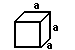(a is the length of the side of each edge of the cube)

In words, the surface area of a cube is the area of the six squares that cover it. The area of one of them is a*a, or a 2 . Since these are all the same, you can multiply one of them by six, so the surface area of a cube is 6 times one of the sides squared.

Example #1:

Find the surface area if the length of one side is 3 cm

Surface area = 6 × a2

Surface area = 6 × 32

Surface area = 6 × 3 × 3

Surface area = 54 cm2
Surface Area of a Cube = 6 a 2

• The total surface area (TSA) of a cuboid is the sum of the areas of its six faces.  That is: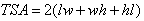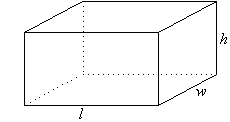#### eg-

Find the total surface area of a cuboid with dimensions 8 cm by 6 cm by 5 cm.

##### Solution: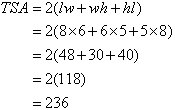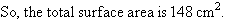Surface area of cuboid = 2lw + 2lh + 2wh = 2(lw + lh + wh)

•Find the total surface area of a cylindrical tin of radius 17 cm and height 3 cm.

##### Solution: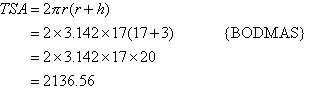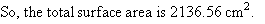###### Summary of Formulas: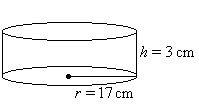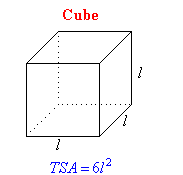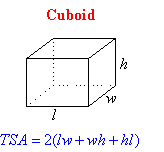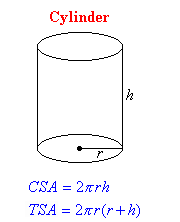• 3

? refers into [ multiply sign ]

• 1

it must be given in your textbook

• 1
What are you looking for?